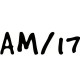Economics Notes (Class 12)

CBSE Class 12 Economics Microeconomics Chapter 4 Theory of Firm Under Perfect Competition

Rate this post

Theory of Firm Under Perfect CompetitionTheory of Firm Under Perfect Competition

Important Notes

1. Perfect Competition A market in which we find perfect competition between a large number of buyers and a large number of sellers of a homogeneous product and the uniform price is called perfect competition market.

2. Features of Perfect Competition
(i) A large number of buyers and sellers
(ii) Homogeneous product
(iii) freedom of entry or exit
(iv) Perfect mobility
(v) Perfect knowledge

3. Price Line The line plotted for different values of output in the output price plane is called price line. In the perfectly competitive market for an individual firm price line and demand curve are the same.

4. Revenue It refers to the money receipts of a firm from the sale of its output.

5. Total Revenue (TR) It is the sum total of revenue derived from the sale of all units of the commodity.
TR= P x Q or AR x Q
or ΣMR
Here, P = Price,Q = Output
AR = Average revenue.MR = Marginal Revenue

6. Average Revenue It is the revenue per unit output sold AR = TR/Q

7. Marginal Revenue: It is the change in total revenue as a result of selling one more (or less) unit of output. MR= TRn – TRn-1 or MR = ΔTR/ΔQ

8. The shape of TR, AR, MR, curves in Perfect Competition
(i) Under Perfect. Competition TR curve in an upward sloping straight line starting from the origin.
(ii) Under Perfect Competition, AR and MR curve is same and || to X-axis.

9. Profit It is the difference between revenue and cost. Profit= (π)= Revenue – Cost

10. Break Even Point: Breakeven for a firm occurs when it is able to cover its all costs of production. Accordingly, break-even point is defined as a situation when TR= TC or AR = AC Under this situation, the firm earns only normal profits.

11. Shutdown Point It occurs when the firm is just able to cover its variable costs, increasing the loss of fixed cost of production. Accordingly shut down point is defined as a situation when TR= TVC or AR= AVC

12. Producer Equilibrium or Profit Maximisation A producer is said to be in equilibrium when he maximises his profits or minimises his losses.
The condition of profit maximisation
(i) MR =MC
(ii) Me is rising or MC should cut MR from below.

13. Profit Maximisation in the Short-run under Perfect Competition
• Condition-1, MR= MC or AR = P
• Condition-2, MC curve should cut the MR= AR curve from below
• Condition-3 P ≥ AVC

14. Short run Supply Curve The supply curve of a firm tells us the quantity of the product that a profit maximising firm is willing to produce at each possible price.

15. Meaning of Supply Supply means the amount of a commodity that firms are able and willing to offer for sale in the market in a given period of time and at a given price.

16. Supply Schedule Tabular statements of the relationship between price and supply of the commodity are called supply schedule.

17. Supply Curve Graphical presentation of the relationship between price and supply of a commodity is called supply curve.

18. Market Supply Curve The market supply curve for a commodity shows the relationship between the price of a given commodity and quantity sellers are inclined to sell.

19. The determinant of Supply Curve
(i) Technological progress
(ii) Input price
(iii) Unit tax

20. The elasticity of Supply it can be defined as a measure of the degree of responsiveness of quantity supplied to changes in the commodity’s own prices.

21. Measurement of Elasticity of Supply Percentage method E =% Change in quantity supplied / % Change in price or ΔQ/ΔP = P/Q
Here, P = Actual Price, Q=  Actual Quantity
ΔP =  Change in Price, ΔQ = Change in Quantity

22. Two Extreme Cases of Elasticity of Supply
(i) Perfect elastic supply (es= ∞)
(ii) Perfect inelastic supply (es = 0)